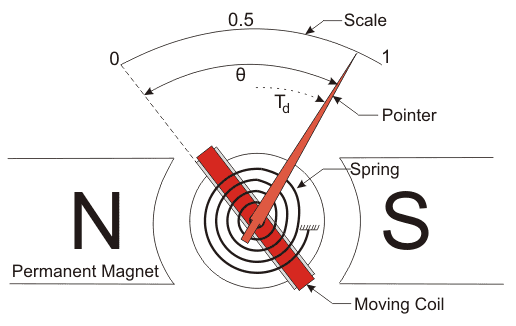# Electrical Measuring Instruments | Types Accuracy Precision Resolution Speed

Basically there are three types of measuring instruments and they are

1. Electrical measuring instruments
2. Mechanical measuring instruments.
3. Electronic measuring instruments.

Here we are interested in electrical measuring instruments so we will discuss about them in detail. Electrical instruments measure the various electrical quantities like electrical power factor, power, voltage and current etc. All analog electrical instruments use mechanical system for the measurement of various electrical quantities but as we know that the all mechanical system has some inertia therefore electrical instruments have a limited time response.

Now there are various ways of classifying the instruments. On broad scale we can categorize them as:

### Absolute Measuring Instruments

These instruments give output in terms of physical constant of the instruments. For example Rayleigh’s current balance and Tangent galvanometer are absolute instruments.

### Secondary Measuring Instruments

These instruments are constructed with the help of absolute instruments. Secondary instruments are calibrated by comparison with absolute instruments. These are more frequently used in measurement of the quantities as compared to absolute instruments, as working with absolute instruments is time consuming.

Another way of classifying the electrical measuring instruments depends on the way they produce the result of measurements. On this basis they can be of two types:

### Deflection Type Instruments

In these types of instruments, pointer of the electrical measuring instrument deflects to measure the quantity. The value of the quantity can be measured by measuring the net deflection of the pointer from its initial position. In order to understand these types of instruments let us take an example of deflection type permanent magnet moving coil ammeter which is shown below:The diagram shown above has two permanent magnets which are called the stationary part of the instrument and the moving part which is between the two permanent magnets that consists of pointer. The deflection of the moving coil is directly proportion to the current. Thus the torque is proportional to the current which is given by the expression Td = K.I, where Td is the deflecting torque.

K is proportionality constant which depends upon the strength of the magnetic field and the number of turns in the coil. The pointer deflects between the two opposite forces produced by the spring and the magnets. And the resulting direction of the pointer is in the direction of the resultant force. The value of current is measured by the deflection angle θ, and the value of K.

### Null Type Instruments

In opposite to deflection type of instruments, the null or zero type electrical measuring instruments tend to maintain the position of pointer stationary. They maintain the position of the pointer stationary by producing opposing effect. Thus for the operation of null type instruments following steps are required:

1. Value of opposite effect should be known in order to calculate the value of unknown quantity.
2. Detector shows the balance and the unbalance condition accurately.

The detector should also have the means for restoring force.
Let us look at the advantages and disadvantages of deflection and null type of measuring instruments:

1. Deflection type of instruments is less accurate than the null type of instruments. It is because, in the null deflecting instruments the opposing effect is calibrated with the high degree of accuracy while the calibration of the deflection type instruments depends on the value of instrument constant hence usually not having high degree of accuracy.
2. Null point type instruments are more sensitive than the Deflection type instruments.
3. Deflection type instruments are more suitable under dynamic conditions than null type of instruments as the intrinsic responses of the null type instruments are slower than deflection type instruments.

Following are the important three functions of the electrical measuring instruments.

### Indicating Function

These instruments provide information regarding the variable quantity under measurement and most of the time this information are provided by the deflection of the pointer. This kind of function is known as the indicating function of the instruments.

### Recording Function

These instruments usually use the paper in order to record the output. This type of function is known as the recording function of the instruments.

### Controlling Function

This is function is widely used in industrial world. In this topic these instruments controls the processes.
Now there are two characteristics of electrical measuring instruments and measurement systems. They are written below:

### Static Characteristics

In these type of characteristics measurement of quantities are either constant or vary slowly with the time. Few main static characteristics are written below:

1. Accuracy:
It is desirable quality in measurement. It is defined as the degree of the closeness with which instrument reading approaches the true value of the quantity being measured. Accuracy can be expressed in three ways

1. Point accuracy
2. Accuracy as the percentage of scale of range
3. Accuracy as percentage of true value.
2. Sensitivity:
It is also desirable quality in the measurement. It is defined as the ratio of the magnitude response of the output signal to the magnitude response of the input signal.
3. Reproducibility:
It is again a desirable quality. It is defined as the degree of the closeness with which a given quantity may be repeatedly measured. High value of reproducibility means low value of drift. Drift are of three types

1. Zero drift
2. Span drift
3. Zonal drift

### Dynamic Characteristics

These characteristics are related with the rapidly changing quantities therefore in order to understand these types of characteristics we are required to study the dynamic relations between the input and the output.

Want To Learn Faster? 🎓
Get electrical articles delivered to your inbox every week.
No credit card required—it’s 100% free.